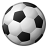# QlikView App Dev

Discussion Board for collaboration related to QlikView App Development.

Announcements
cancel
Showing results for
Did you mean:Creator

## Ignore Year selection, within chart or table

Afternoon all,

As per the attached, you'll see I've created two tables.

One showing the total and the other showing what's older than 60 days.

Rightly so, difference being the two months at the bottom, have been taken away and I get the correct answer.

However, when you select any year... the older than 60 days table changes too.

As you can see below, I've put in the Year field (highlighted in red)... which I thought would ignore any user selection, of that field.

sum({<[Year_YYYY]=>} Unbilled_WIP_Amount)
-
Sum({<[Year_YYYY]=,[Period_Sort]={">=\$(=max({\$}[Period_Sort])-1)<=\$(=max({\$}[Period_Sort]))"}>} Unbilled_WIP_Amount)

The issue seems to be coming from the second part of the calculation.

Any ideas, where I'm going wrong?

Help much appreciated, as always.

1 Solution

Accepted SolutionsMVP

Try this:

Sum({<[Year_YYYY]=>} Unbilled_WIP_Amount)
-
Sum({<[Year_YYYY]=, [Period_Sort]={">=\$(=max({<[Year_YYYY]=>}[Period_Sort])-1)<=\$(=max({<[Year_YYYY]=>}[Period_Sort]))"}>} Unbilled_WIP_Amount
)

4 RepliesMVP

Try this:

Sum({<[Year_YYYY]=>} Unbilled_WIP_Amount)
-
Sum({<[Year_YYYY]=, [Period_Sort]={">=\$(=max({<[Year_YYYY]=>}[Period_Sort])-1)<=\$(=max({<[Year_YYYY]=>}[Period_Sort]))"}>} Unbilled_WIP_Amount
)Employee

This is the right expression not dependent on Year:

sum({<[Year_YYYY]=>} Unbilled_WIP_Amount)
-

Sum({<[Year_YYYY]=,[Period_Sort]={">=\$(=max({\$ <[Year_YYYY]=>}[Period_Sort])-1)<=\$(=max({\$ <[Year_YYYY]=>}[Period_Sort]))"}>} Unbilled_WIP_Amount)Partner

Hi,

Beat me to the post again. But yer like Sunny suggests. You have set analysis within set analysis so you need to exclude the year selection from each.

MarkCreator
Author

thanks for replying everyone, most helpful as alwaysSet analysis, within a set analysis... genius this tool and makes sense

Nice one, have a good day all